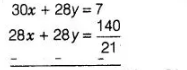# A motorboat can travel 30 km upstream

Question:

A motorboat can travel 30 km upstream and 28 km downstream in 7 h. It can travel 21 km upstream and return in 5 h. Find the speed of the boat in still

water and the speed of the stream.

Solution:

Let the speed of the motorboat in still water and the speed of the stream are u km/h and v km/h, respectively.

Then, a motorboat speed in downstream = (u + v) km/h and a motorboat speed in upstream = (u -v) km/h.

Motorboat has taken time to travel 30 km upstream,

$t_{1}=\frac{30}{u-v} h$

and motorboat has taken time to travel $28 \mathrm{~km}$ downstream,

$t_{2}=\frac{28}{u+v} \mathrm{~h}$

By first condition, a motorboat can travel $30 \mathrm{~km}$ upstream and $28 \mathrm{~km}$ downstream in $7 \mathrm{~h}$ i.e.,

$t_{1}+t_{2}=7 \mathrm{~h}$

$\Rightarrow$ $\frac{30}{u-v}+\frac{28^{2}}{u+v}=7$ ...(i)

Now, motorboat has taken time to travel $21 \mathrm{~km}$ upstream and return i.e.. $t_{3}=\frac{21}{u-v}$.

[for ūpstream]

and $t_{4}=\frac{21}{u+v}$ [for downstream]

By second condition, $t_{4}+t_{3}=5 \mathrm{~h}$

$\Rightarrow$ $\frac{21}{u+v}+\frac{21}{u-v}=5$ ...(ii)

Let $x=\frac{1}{u+v}$ and $y=\frac{1}{u-v}$

Eqs. (i) and (ii) becomes $\quad 30 x+28 y=7$ ... (iii)

and $\quad 21 x+21 y=5$

$\Rightarrow$ $x+y=\frac{5}{21}$ ...(iv)

Now, multiplying in Eq. (iv) by 28 and then subtracting from Eq. (iii), we get$2 x=7-\frac{20}{3}=\frac{21-20}{3}$

$\Rightarrow$ $2 x=\frac{1}{3} \Rightarrow x=\frac{1}{6}$

On putting the value of $x$ in Eq. (iv), we get

$\frac{1}{6}+y=\frac{5}{21}$

$\Rightarrow$ $y=\frac{5}{21}-\frac{1}{6}=\frac{10-7}{42}=\frac{3}{42} \Rightarrow y=\frac{1}{14}$

$\because$ $x=\frac{1}{u+v}=\frac{1}{6} \Rightarrow u+v=6$ ..(v)

and $y=\frac{1}{u-v}=\frac{1}{14}$

$\Rightarrow \quad u-v=14$ $\ldots$ (vi)

Now, adding Eqs. (v) and (vi), we get

$2 u=20 \Rightarrow u=10$

On putting the value of $u$ in Eq. (v), we get

$10+v=6$

$\Rightarrow \quad v=-4$

Hence, the speed of the motorboat in still water is 10 km/h and the speed of the stream 4 km/h.